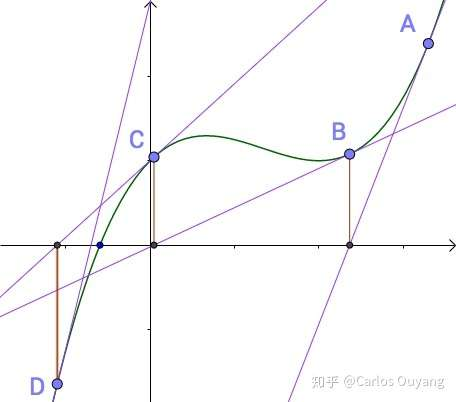# LeetCode 69 题

## 1.题目要求

输入: 4



输入: 8

由于返回类型是整数，小数部分将被舍去。

## 2.初次尝试

 1 class Solution {
2     public int mySqrt(int x) {
3         for(int i = 0; i <= x; i++) {
4             if(i * i >= x){
5                 if(i * i == x)
6                     return i;
7                 else
8                     return i - 1;
9             }
10         }
11         return 0;
12     }
13 }

1 class Solution {
2     public int mySqrt(int x) {
3         return (int)Math.sqrt(x);
4     }
5 }

## 3.牛顿迭代法## 4.牛顿迭代法求平方根

 1 class Solution {
2     public int mySqrt(int x) {
3         if(x == 1)
4             return 1;
5         double _x = x >> 1;
6         double _y = _x * _x - x;
7         double a = (-_y + 2 * _x * _x) / 2 / _x;
8         while(_y > 0.1 || _y < -0.1){
9             _x = a;
10             _y = _x * _x - x;
11             a = (-_y + 2 * _x * _x) / 2 / _x;
12         }
13         return (int)_x;
14     }
15 }

## 5.简化

 1 class Solution {
2     public int mySqrt(int x) {
3         if(x == 1 || x == 0)
4             return x;
5         int a = x >> 1;
6         while(a > x / a){
7             a = (a + x/a) / 2;
8         }
9         return a;
10     }
11 }

posted @ 2019-05-06 20:13  Carlos_Ouyang  阅读(337)  评论(0编辑  收藏